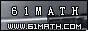Today is . WELCOME: huohai3983/44663  Home | Reg Login | All user | setHomePage | BookmarkHome | Math Test Online | Addition | Subtraction | Multiplication | Division | Time | Worksheet(JPG/PDF) | CAI | Flash Games |Recommendation articleArticle searchHome--maths--Fourth Grade--Properties of Addition

There are four mathematical properties which involve addition. The properties are the commutative, associative, additive identity and distributive properties.

Commutative property: When two numbers are added, the sum is the same regardless of the order of the addends. For example 4 + 2 = 2 + 4

Associative Property: When three or more numbers are added, the sum is the same regardless of the grouping of the addends. For example (2 + 3) + 4 = 2 + (3 + 4)

Additive Identity Property: The sum of any number and zero is the original number. For example 5 + 0 = 5.

Distributive property: The sum of two numbers times a third number is equal to the sum of each addend times the third number. For example 4 * (6 + 3) = 4*6 + 4*3

#### What property of addition is shown?

2006-10-24 22:33:26
Viewed 6371 times CloseGreatmathsites